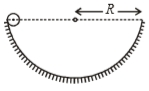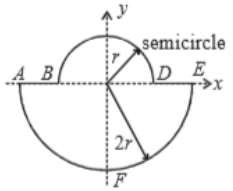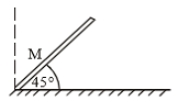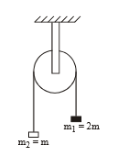In the figure shown, a small solid spherical ball of mass 'm' can move without sliding in a fixed semicircular track of radius R in vertical plane. It is released from the top. The resultant force on the ball at the lowest point of the track is :1.

2.

3.

4. zero

Concept Questions :-

Rolling motion
High Yielding Test Series + Question Bank - NEET 2020

Difficulty Level:

A uniform thin rod is bent in the form of closed loop ABCDEFA as shown in the figure. The ratio of inertia of the loop about x-axis to that about y-axis is:1.  >1

2.  <1

3.  =1

4.  =1/2

Concept Questions :-

Moment of inertia
High Yielding Test Series + Question Bank - NEET 2020

Difficulty Level:

Four spheres of diameter 2a and mass M are placed with their centres on the four corners of a square of side b. Then the moment of inertia of the system about an axis about one of the sides of the square is

(1) $M{a}^{2}+2M{b}^{2}$

(2) $M{a}^{2}$

(3) $M{a}^{2}+4M{b}^{2}$

(4) $\frac{8}{5}M{a}^{2}+2M{b}^{2}$

Concept Questions :-

Moment of inertia
High Yielding Test Series + Question Bank - NEET 2020

Difficulty Level:

A body of mass (m) elastically collides with another stationary body of mass 3m. The fractional loss of K.E. of the body is:

1.  $\frac{8}{9}$

2.  $\frac{3}{4}$

3.  $\frac{3}{5}$

4.  $\frac{4}{7}$

Concept Questions :-

Linear momentum
High Yielding Test Series + Question Bank - NEET 2020

Difficulty Level:

A hollow sphere is rolling without slipping on the inclined plane. The minimum coefficient of friction is :

1. $\frac{2}{5}\mathrm{tan}\theta$

2. $\frac{2}{3}\mathrm{tan}\theta$

3. $\frac{3}{4}\mathrm{tan}\theta$

4. $\frac{5}{7}\mathrm{tan}\theta$

Concept Questions :-

Rolling motion
High Yielding Test Series + Question Bank - NEET 2020

Difficulty Level:

A body of mass (m) is projected with velocity v0 at an angle of projection $\mathrm{\theta }$. The magnitude of angular momentum about origin after time t from projection is :

1. Increase with time

2. decrease with time

3. independent of time

4. none of these

Concept Questions :-

Angular momentum
High Yielding Test Series + Question Bank - NEET 2020

Difficulty Level:

A body of mass m slides down an incline and reaches the bottom with a velocity v. If the same mass were in the form of a ring which rolls down this incline, the velocity of the ring at bottom would have been

(1) v

(2)

(3) $\left(1/\sqrt{2}\right)v$

(4) $\left(\sqrt{2/5}\right)v$

Concept Questions :-

Rolling motion
High Yielding Test Series + Question Bank - NEET 2020

Difficulty Level:

A rod is released at an angle 45$°$ to the horizontal as shown. If its length is L, its angular acceleration about its foot at ground is initially1. $\frac{3\mathrm{g}}{2\mathrm{L}}$

2. $\frac{3\mathrm{g}}{\sqrt{2}\mathrm{L}}$

3. $\frac{3\mathrm{g}}{2\sqrt{2}\mathrm{L}}$

4. $\frac{6\mathrm{g}}{\sqrt{2}\mathrm{L}}$

Concept Questions :-

Torque
High Yielding Test Series + Question Bank - NEET 2020

Difficulty Level:

From a disc of mass M and radius '2a', a concentric disc of radius 'a' is cut out. The moment of inertia of remaining disc is

1.

2.

3.

4.  $\frac{3}{2}{\mathrm{Ma}}^{2}$

High Yielding Test Series + Question Bank - NEET 2020

Difficulty Level:

The moment of inertia of pulley is I and its radius is R. The string doesn't slips on the pulley, as system is released, the acceleration of the system is(1) $\frac{g}{\left(3+\frac{I}{m{R}^{2}}\right)}$

(2) $\frac{g}{\left(1+\frac{I}{m{R}^{2}}\right)}$

(3) $\frac{3g}{\left(1+\frac{I}{m{R}^{2}}\right)}$

(4) $\frac{3g}{\left(2+\frac{I}{m{R}^{2}}\right)}$

Concept Questions :-

Torque